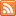# Content Tagged “LO 6.5”## Conditional Probability and Independence

Published: Jul 28th, 2012

Independent Events Multiplication Rule for Independent Events (Rule Six) Conditional Probability (Rule Seven) Independent Events (Part 2) General Multiplication Rule (Rule Eight) Let’s Summarize CO-6: Apply basic concepts of probability, random […]## Basic Probability Rules

Published: Jul 28th, 2012

Introduction Rules of Probability Probability Rule One (For any event A, 0 ≤ P(A) ≤ 1) Probability Rule Two (The sum of the probabilities of all possible outcomes is 1) Probability Rule […]## Introduction to Probability

Published: Jul 28th, 2012

Probability is Not Always Intuitive Need a Laugh? What is Probability? Probability is the Likelihood of Something Happening Notation Determining Probability To Summarize So Far Relative Frequency Let’s Summarize CO-6: Apply […]